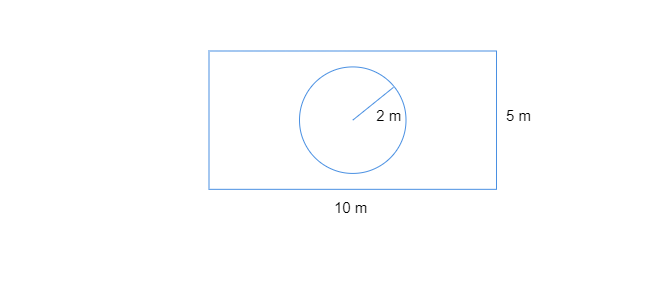$(i)$ the area of the whole land
$(ii)$ the area of the flower bed
$(iii)$ the area of the lawn excluding the area of the flower bed
$(iv)$ the circumference of the flower bed." ">

# The adjoining figure represents a rectangular lawn with a circular flower bed in the middle. Find:$(i)$ the area of the whole land $(ii)$ the area of the flower bed$(iii)$ the area of the lawn excluding the area of the flower bed$(iv)$ the circumference of the flower bed."

$(i)$. Length of the lawn$= 10\ m$

Breadth of the lawn$= 5\ m$

Area of the lawn$= l\times b$

$=10m\times5m=50\ m^2$

$(ii)$.  Area of the circular flower bed$= \pi r^2$

$\frac{22}{7}\times2\times2=\frac{88}{7}m^2=12.57\ m^2$

$(iii)$.  Area of the lawn excluding the area of the flower bed

$=50m^2-\frac{88}{7}\ m^2$

$=\frac{350-88}{7}\ m^2=\frac{262}{7}\ m^2=37.43\ m^2$

$(iv)$. Circumference of the flower bed$= 2\pi r^2$

$=2\times\frac{22}{7}\times2=12.56\ m$

Updated on: 10-Oct-2022

56 Views